# High School Math : How to find the length of the side of a trapezoid

## Example Questions

### Example Question #1 : Trapezoids

The following quadrilaterals are similar. Solve for.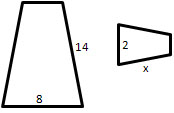(Figure not drawn to scale).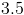Explanation:

When polygons are similar, the sides will have the same ratio to one another. Set up the appropriate proportions.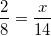Cross multiply.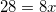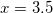### All High School Math Resources